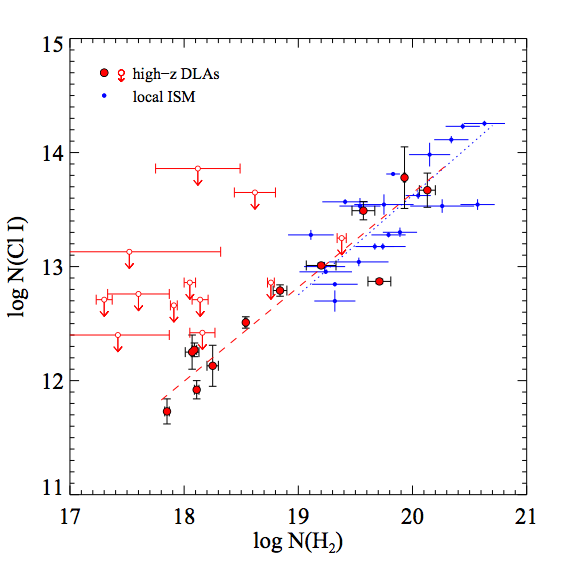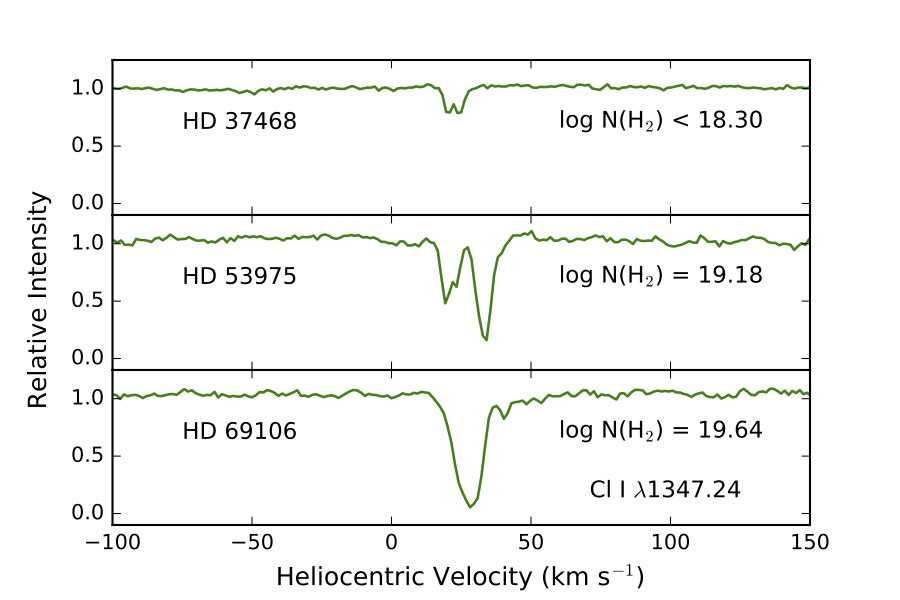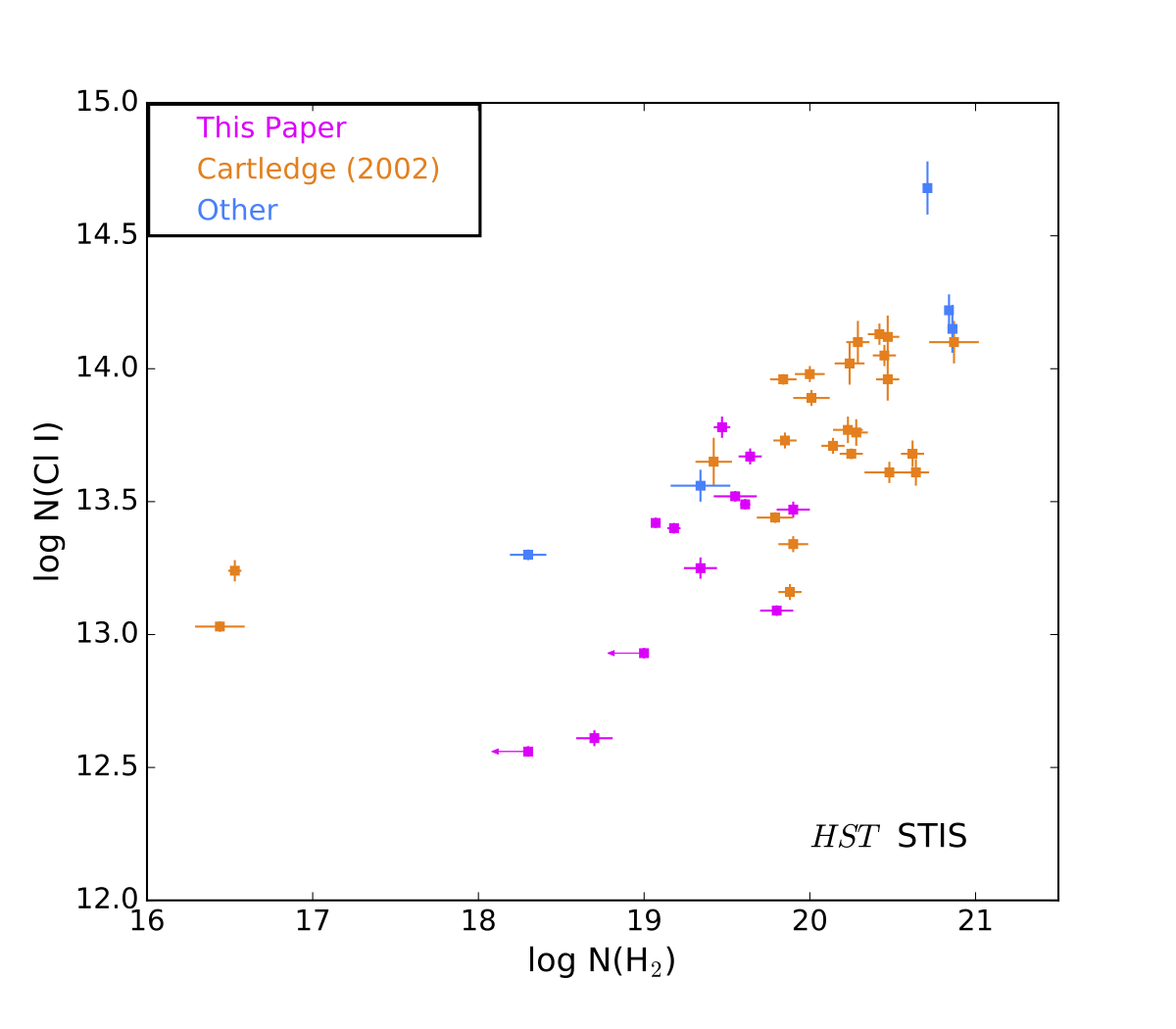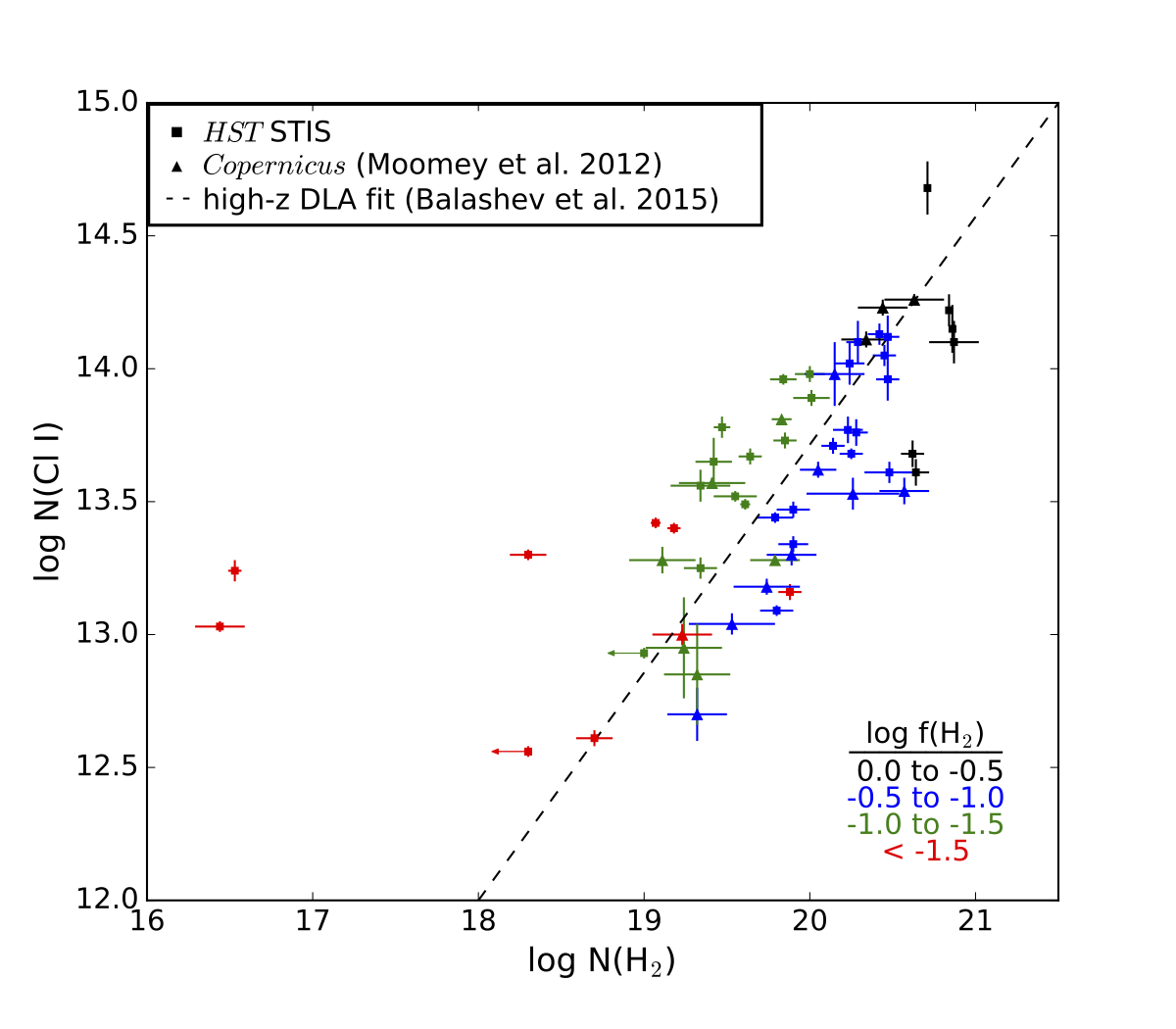Hello, my name is Valerie Becker, and this summer I participated in a Research Experience for Undergraduates (REU) at Northwestern University. I am currently pursuing an undergraduate physics major at Southern Illinois University Edwardsville. I plan to attend graduate school in physics or astronomy after I graduate. I spend my time doing STEM outreach and organizing events for SIUE's Physics Club. In my free time I enjoy juggling, folding origami, and solving fun problems.

On this page is an outline of the research I conducted this past summer with CIERA. I will also be presenting this information in poster form at the 229th AAS conference in Grapevine, Texas.

# HST STIS Observations of Interstellar Chlorine

This summer, I worked on this project under the direction of Dr. David M. Meyer of Northwestern University. In these nine weeks I learned about observational astronomy and data reduction methods. Specific areas of astronomy in this project include interstellar medium, interstellar chlorine, and the Hubble Space Telescope (HST). The technical skills I worked on were using IRAF, FITS6P, and mastering Python.

### AAS Abstract:

Among the dominant ions of abundant elements in the diffuse interstellar medium, only chlorine (Cl II) has a rapid exothermic reaction with molecular hydrogen (H$_2$) that should lead to the dominance of its atomic form (Cl I) in clouds where most of the hydrogen is in H$_2$. We present the results of an archival study of the interstellar Cl I $\lambda$1347.24 absorption observed at high spectral resolution toward 41 stars with the Space Telescope Imaging Spectrograph (STIS) onboard the Hubble Space Telescope (HST). Our key goals in this survey are to explore the relationship between interstellar N(Cl I) and N(H$_2$) with a larger sample and a larger N(H$_2$) range (16.44 < log N(H$_2$) < 20.87) than the Copernicus interstellar survey of Moomey et al. (2012). We additionally contrast it with the high-z QSO damped Lyman-alpha system (DLA) findings of Balashev et al. (2015). We find that for log N(H$_2$) > 19.0, the HST STIS sample is consistent with the Copernicus data and high-z DLA samples in indicating a linear trend of increasing N(Cl I) with increasing N(H$_2$). Furthermore, all of the interstellar sightlines with log f(H$_2$) > -0.5 have log N(Cl I) > 13.5, and those with log f(H$_2$) < -1.5 have log N(Cl I) < 13.5, where $f(H_2) = \frac{2N(H_2)}{2N(H_2) + N(HI)}$ is the fractional amount of H$_2$ in H. Consequently, observations of interstellar Cl I can potentially trace the H$_2$ fraction of the "CO-dark" gas marking the transition between diffuse atomic and dense CO molecular clouds.

Author(s): Valerie R. Becker1, 2, Cody Dirks2, David M. Meyer2, Stefan I.B. Cartledge3
Institution(s): 1. Department of Physics, Southern Illinois University Edwardsville 2. CIERA, Department of Physics and Astronomy, Northwestern University 3. Department of Physical Sciences, MacEwan University

## Motivations

Among the dominant ions of abundant elements in the diffuse interstellar medium, only chlorine (Cl II) has a rapid exothermic reaction with molecular hydrogen (H$_2$) that should lead to the dominance of its atomic form (Cl I) in clouds where most of the hydrogen is in H$_2$ (Jura 1974; Jura & York 1978; Harris & Bromage 1984). Recently, Moomey et al. (2012) have reanalyzed Copernicus UV observations of interstellar Cl I and Cl II in 21 sightlines with 19.11 < log N(H$_2$) < 20.63 and found a clear correspondence between the column densities of Cl I and H$_2$. Balashev et al. (2015) have extended this work to high-redshift QSO damped Ly$\alpha$ systems (DLAs) and detected Cl I absorption in 12 DLAs with 17.85 < log N(H$_2$) < 20.13. As shown in Fig. 1, their DLA finding of a significant linear relationship between N(Cl I) and N(H2) is remarkably consistent with the interstellar Moomey et al. (2012) result. The clear implication of Fig. 1 is that the abundance of interstellar Cl I can be used to estimate the volume of H$_2$ in interstellar and extragalactic gas clouds.Figure 1. A comparison of column densities Cl I vs H$_2$ in interstellar clouds (blue) and extragalactic clouds (red) with corresponding trend lines from Balashev et al. (2015).

In this project, we describe the findings of an archival study of the interstellar Cl I $\lambda$1347.24 absorption observed at high spectral resolution toward 41 stars with the Space Telescope Imaging Spectrograph (STIS) onboard the Hubble Space Telescope (HST). Our key goals in this survey are to investigate the connection between interstellar N(Cl I) and N(H$_2$) with a larger sample and a larger N(H$_2$) range (16.44 < log N(H$_2$) < 20.87) than that of Moomey et al. (2012) and contrast it with the DLA findings of Balashev et al. (2015).

## The Data

Among the 41 HST STIS measurements of interstellar N(Cl I) described here, only five have previously been reported in the literature (Sonnentrucker et al. 2006; Welty et al. 1999; Welty 2007). An additional 24 N(Cl I) measurements have been taken from the PhD dissertation of Stefan Cartledge (2002) on interstellar elemental abundances. The remaining 12 sightlines were chosen to add more low N(H$_2$) (log N(H$_2$) < 20.0) cases to the sample. Their N(Cl I) values were measured from high-resolution (R $\approx$ 110,000 or R $\approx$ 200,000) STIS spectra downloaded from the HST archive. Fig. 2 illustrates the Cl I $\lambda$1347.24 spectra for three of these stars as a function of increasing N(H$_2$).Figure 2. A sample of HST Cl I spectra used for profile-fitting.

Column densities were measured from these Cl I absorption lines using the FITS6P iterative profile-fitting program (Welty et al. 1994) and the corresponding interstellar Mg II $\lambda\lambda$1239.93, 1240.40 doublet to help correct for the modest line saturation. Fig. 3 compares the Cl I and H$_2$ column densities for all of the 41 sightlines in our HST STIS sample. The N(H$_2$) values have been measured from far-UV spectra by Burgh et al. (2007), Bohlin et al. (1978), Cartledge et al. (2008), Cartledge et al. (2004), Jenkins (2009), Olivera & Hebrard (2006), Rachford et al. (2002).Figure 3. Cl I vs H$_2$ column densities color coded with respect to each HST STIS Cl I source.

## Conclusions

Fig. 4 compares the Cl I and H$_2$ column densities for both the HST STIS and Moomey et al. (2012) Copernicus interstellar samples with the Balashev et al. (2015) fit to the high-z DLA data. The interstellar data points are color-coded in terms of the log f(H$_2$) values of their sightlines where $f(H_2) = \frac{2N(H_2)}{2N(H_2) + N(HI)}$ - the fractional abundance of H in H$_2$.Figure 4. Cl I vs H$_2$ column densities color-coded based on values of log f(H$_2$). The high-z DLA fit is plotted from Fig. 1 for comparison.

Based on the results summarized in Fig.4, we can draw the following conclusions:
1. For log N(H$_2$) > 19.0, the HST STIS sample is consistent with the Copernicus and high-z DLA samples in revealing a linear trend of increasing N(Cl I) with increasing N(H$_2$).
2. For log N(H$_2$) < 19.0, the STIS interstellar sample does not exhibit the linear relationship between N(Cl I) and N(H$_2$) found in the high-z DLA sample.
3. All of the interstellar sightlines with log f(H$_2$) > -0.5 have a log N(Cl I) > 13.5.
4. All of the interstellar sightlines with log f(H$_2$) < -1.5 have a log N(Cl I) < 13.5.
Consequently, observations of interstellar Cl I can potentially trace the H$_2$ fraction of the "CO-dark" gas marking the transition between diffuse atomic and dense CO molecular clouds.

## Acknowledgements & References

### Acknowledgements

This material is based upon work supported by the National Science Foundation under Grant No. AST-1359462, a Research Experiences for Undergraduates (REU) grant awarded to CIERA at Northwestern University. Any opinions, findings, and conclusions or recommendations expressed in this material are those of the author(s) and do not necessarily reflect the views of the National Science Foundation.